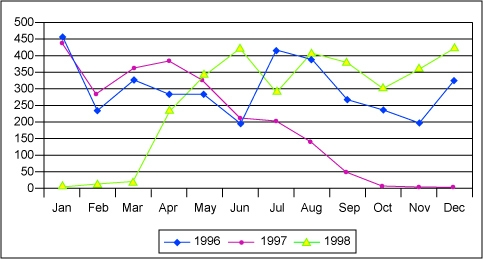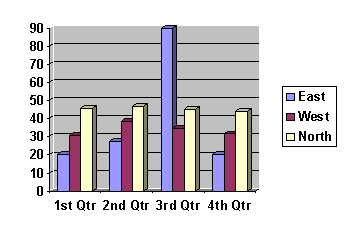# Interpreting and Measuring Data

## Lesson Objective

In this lesson we will review measurement and calculation within science.

## Data

Data is the information collected during an experiment. This information could be weight, temperature, pH, or any other scientific investigative material. Since we have this information and we conducted the experiment, we understand the data we collected. However, how do we explain this data to someone else? How do we display this data so it can be easily read and interpreted? Many scientists use graphs to showcase data. There are many types of graphs or charts that may be utilized: line, bar, pie, diagrams, and sometimes even models or maps. In order to make it possible for our audience to understand our data, a legend and key for all of these types of data displays should be included. The most commonly used charts or graphs are a line graph, bar graph, and pie chart.

Line graphs are used to summarize how two pieces of information are related and how they vary depending on one another.Example of a line graph used correctly to show the large impact of the 1997-1998 El Niño Southern Oscillation effect on precipitation on the island of New Guinea.

Bar graphs
consist of an axis and a series of labeled horizontal or vertical bars that show different values for each bar. The numbers along a side of the bar graph are called the scale.This graph depicts how many sales were made by each division over a time period denoted by quarters. For this bar graph the independent variable is plotted on the x-axis and the dependent variable is plotted on the y-axis. For bar graphs, the variables can be designated on either axis.

Pie charts are used to display the sizes of parts that make up some whole. A pie chart is a circle graph divided into pieces, each displaying the size of some related piece of information.This pie chart accurately shows the different numbers of frog clutches found in 1998. Each category is a separate micro-habitat where the frogs live and lay their eggs.

Sometimes data must be depicted utilizing numbers. All numbers must be consistent. To accomplish this task, scientists use scientific notation.